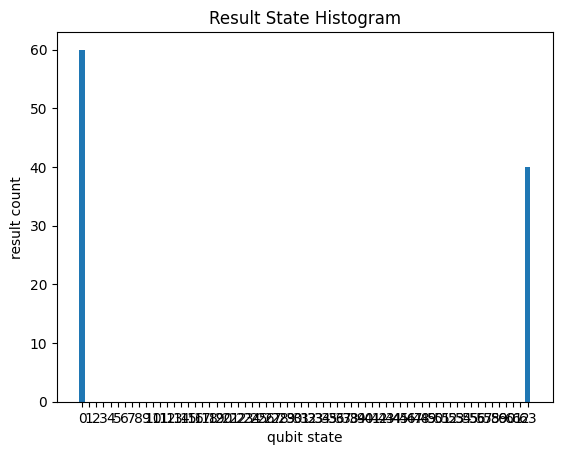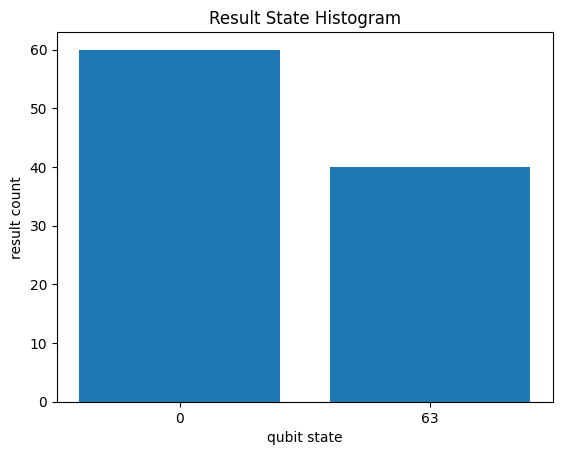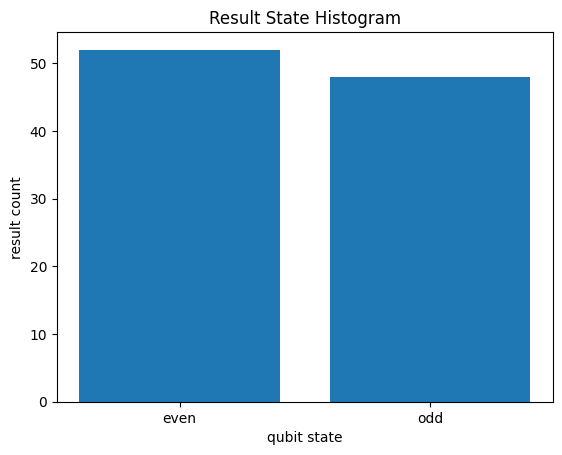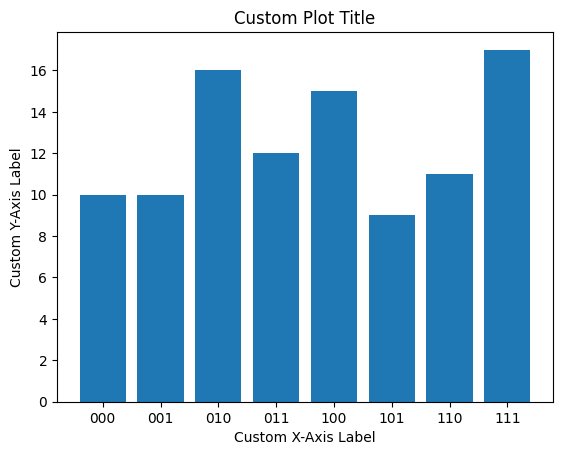# State Histograms

State Histograms are useful to visualize the output of running a quantum circuit. For details on how to create and run your own quantum circuits, please see Cirq basics

``````try:
import cirq
except ImportError:
print("installing cirq...")
!pip install --quiet cirq
print("installed cirq.")
import cirq
import matplotlib.pyplot as plt
``````

### Basic usage

``````q = cirq.LineQubit.range(4)
circuit = cirq.Circuit([cirq.H.on_each(*q), cirq.measure(*q)])
result = cirq.Simulator().run(circuit, repetitions=100)
_ = cirq.plot_state_histogram(result, plt.subplot())
plt.show()
``````### Plotting circuits with sparse output

If the results have sparse output, you can use the `result.histogram` to compute the histogram and plot only states that have non-zero sample count. For example:

#### Default plot

The default call to `cirq.plot_state_histogram` would plot all possible output states (including 0's). This can get messy, especially if the output is sparse.

``````q = cirq.LineQubit.range(6)
circuit = cirq.Circuit([cirq.H(q), [cirq.CNOT(q, q[i]) for i in range(1, 6, 1)], cirq.measure(*q, key='measure_all')])
result = cirq.Simulator().run(circuit, repetitions=100)
_ = cirq.plot_state_histogram(result, plt.subplot())
plt.show()
``````#### Sparse plots

To plot only non-zero entries in the histogram, you can first compute a histogram using `result.histogram()` and pass its result to `cirq.plot_state_histogram`

``````histogram = result.histogram(key = 'measure_all')
_ = cirq.plot_state_histogram(histogram, plt.subplot())
plt.show()
``````### Histogram for processed results.

`result.histogram()` also allows you to pass a `fold_func` parameter that can be used to process the sampled measurement results and convert to a countable value to generate a histogram.

As an example, suppose you want to plot a histogram of the parity of the number of times a `1` occurs in a measurement result. We can do this using `fold_func` as follows:

``````def count_ones(bits):
return 'even' if sum(bits) % 2 == 0 else 'odd'

q = cirq.LineQubit.range(6)
# Uniform superposition over all 2 ** 6 states.
circuit = cirq.Circuit([cirq.H.on_each(*q), cirq.measure(*q, key='measure_all')])
result = cirq.Simulator().run(circuit, repetitions=100)
# Create a customized histogram by processing the measurement results using `fold_func`.
custom_histogram = result.histogram(key = 'measure_all', fold_func=count_ones)
# Plot the custom histogram using cirq.plot_state_histogram
_ = cirq.plot_state_histogram(custom_histogram, plt.subplot())
plt.show()
``````### Modifying plot properties

You can pass additional arguments to the `cirq.plot_state_histogram` method to modify plot properties like `title`, `xlabel`, `ylabel` and `tick_label`. For example:

``````def binary_labels(num_qubits):
return [bin(x)[2:].zfill(num_qubits) for x in range(2 ** num_qubits)]

q = cirq.LineQubit.range(3)
circuit = cirq.Circuit([cirq.H.on_each(*q), cirq.measure(*q)])
result = cirq.Simulator().run(circuit, repetitions=100)
_ = cirq.plot_state_histogram(result, plt.subplot(), title = 'Custom Plot Title', xlabel = 'Custom X-Axis Label', ylabel = 'Custom Y-Axis Label', tick_label=binary_labels(3))
plt.show()
``````[{ "type": "thumb-down", "id": "missingTheInformationINeed", "label":"Missing the information I need" },{ "type": "thumb-down", "id": "tooComplicatedTooManySteps", "label":"Too complicated / too many steps" },{ "type": "thumb-down", "id": "outOfDate", "label":"Out of date" },{ "type": "thumb-down", "id": "samplesCodeIssue", "label":"Samples / code issue" },{ "type": "thumb-down", "id": "otherDown", "label":"Other" }]
[{ "type": "thumb-up", "id": "easyToUnderstand", "label":"Easy to understand" },{ "type": "thumb-up", "id": "solvedMyProblem", "label":"Solved my problem" },{ "type": "thumb-up", "id": "otherUp", "label":"Other" }]